# Quibbling Over Qubits

## A prequel to the quantum computer revolution

Farnam Street recently had a good post about the “Feynman Technique” for learning a subject, with a key step being that you should try to “teach it to a toddler” (i.e. writing out a summary using simple language). In that spirit I’ll try to use this post to tackle the burgeoning field of quantum computers. A computer scientist I am not but have had some (hobbyist) interest in the field for a little while, although this will be the first that I’ve attempted to actually put concepts down to pen and paper outside of a few stray tweets. It is rare that a paradigm shift this significant is so obvious in advance — with all of the talk of Moore’s Law reaching limits of nanoscale transistor fabrication in the coming years quantum computers will allow us to step outside of the law for at least some specific applications. The field has attracted interest of physicists and computer scientists at least since Feynman’s (the same) call to action in 1981 with his talk “Simulating Physics with Computers” or Deutsch’s 1985 paper “Quantum theory, the Church-Turing principle and the universal quantum computer” although the technology for building actual working machines is just now starting to catch up to the hype (so far only of limited scale). There are a few tech firms working hard to realize the potential of quantum computation and I will touch on that briefly, but the goal of this post is primarily to focus on key concepts for building a foundational technical vocabulary. While I will try to provide links for key terms and trade the more arcane aspects of notations for plain English where possible, anyone attempting to read through this post is encouraged to make liberal use of either wikipedia or google for supplement of concepts that require further explanation.

1. What is a qubit?

The simplest way to define a quantum computer, and perhaps the aspect that most often makes it into popular press accounts, is the use of qubits instead of bits for elementary building blocks of computation. While a classical computer’s bits, made of transistors, are binary (meaning two-state e.g. on/off or 1/0), a quantum computer’s equivalent building block, qubits, operates in a quantum superposition of the 1 and 0 states which will collapse to either a value of 1 or 0 upon a measurement.

For this discussion I will mostly abstract out the physical manifestation of the qubit and gate architecture and instead focus on the underlying principles of operation, however it is worth a brief overview of some of the key architectures competing for adoption.

The description of a qubit’s state is a little more complicated with a much wider range of values than a classical bit. To describe the quantum superposition of a qubit requires complex numbers, for example a single qubit’s quantum superposition ψ (a common notation for a superposition, the Greek symbol Psi) could be described as ψ=a|0>+b|1>, where a and b are any combination of complex numbers a and b subject to the constraint that |a|²+|b|²=1, with the absolute value of the complex number a squared (|a|²) is equal to the probability that a measurement of the qubit’s state results in the value 0, similarly the absolute value of the complex number b squared (|b|²) is equal to the probability that a measurement of the qubit’s state results in the value 1, and hence the sum of those two probabilities has to sum to 1 (meaning there is exactly a 100% probability of the measured state of a single qubit being either 1 or 0, or using the “bra-ket” notation the states |0> or |1>). Of course we can’t know the actual values of the superposition ψ with a single measurement of a qubit because each measurement will collapse the state to either of the two values under superposition and we lose all of the probabilistic information, but given enough measurements we can attempt to estimate probabilities — or alternatively if we create a known state of ψ prior to a series of transformations through defined quantum gates then we might know the full representation of the resulting state ψ. An easy way to visualize the range of possible values for a qubit’s superposition between the states |0> and |1> is through what is known as a Bloch Sphere.

A few comments about the Bloch Sphere. First the equivalent formation for a classical computer would just be the two points |0> and |1>, which are shown here as to top and bottom points of the sphere along the z axis.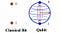The image on the left also represents the potential states of a qubit after a measurement causes the superposition to collapse.

If the superposition ψ where to fall exactly on the point |0> at the top of the z axis for instance there would be a 100% probability of a measurement finding a qubit in state 0. Note that the positions of |0> and |1> are arbitrary, if we wanted we could change our basis of measurement and notation to any two points on opposite ends of the sphere. Another common basis besides |0> and |1> are the states |+> and |->, related to our original basis by the formulas |+>=(1/√2)|0>+(1/√2)|1> and |->=(1/√2)|0>-(1/√2)|1>. This +/- basis is notable because both represent a 50% probability of a measurement revealing either 0 or 1 (i.e. |(1/√2)|²=1/2=50%). As we start subjecting a qubit’s superposition to transformation around the bloch sphere by applying the various quantum gates we may find the alternate measurement and notation basis of |+> and |-> more appropriate, just keep in mind that these notation basis are arbitrary and while it will change the values of a & b to change the basis of notation it won’t change the location on the bloch sphere. This is worth restating for clarity. When a qubit is subject to transformations by applying a quantum gate, it results in some kind of rotation around the various axis of the bloch sphere, thus changing the probability of a measurements collapse to states of |0> or |1> (or depending on basis of measurement perhaps another such as |+> or |->). Keep in mind that the bloch sphere is a two dimensional representation for the state of only a single qubit (this sphere is two dimensional instead of three dimensional because we are restricted to its surface and it is a sphere and not a circle because of the numbers are complex, note that any point on the sphere can be identified with just two variables such as the two angles θ and φ shown in the graphic). As we introduce additional qubits to the system the resulting combined superposition would require a shape of additional dimensions and hence not really as useful for visualization purposes (for example for a two qubit superposition the combined superposition would be described as ψ=a|00>+b|01>+c|10>+d|11>, subject to the restriction that |a|²+|b|²+|c|²+|d|²=1, or in other words |a|² gives the probability that a measurement finds both qubits in the 0 state, |b|² gives the probability of a measurement finding the first qubit in the 0 state and the second qubit in the 1 state, etc.)

Often a quantum computer vendor will report on the number of qubits that are achieved as a measure of their scale. As the Hilbert space (dimensionality) of a quantum computer’s state climbs exponentially with the number of qubits as 2^n this is an important metric. However in evaluating the number of qubits it is important to keep in mind the difference between a functioning logical qubit and a physical qubit. The superimposed state of a physical qubit is extremely fragile, and depending on the architecture, coherence can likely only be maintained for minute fractions of a second, thus there is a demanding need for error correction, comparable to although not identical as the error correction used in a classical computer. These errors cause the ideal shape of the Bloch Sphere to deform in different ways based on the channels of error (i.e. bit flips, phase flips, depolarizing, amplitude dampening, or phase dampening) and their rate. In order to achieve suitable quantum error correction an architecture may have to expend multiple physical qubits to construct a resulting single functioning logical qubit. A key enabler of future more scalable quantum computing architectures will be their ability to maintain their coherence for extended periods and thus reduce the amount of error correction required.

2. What is a quantum gate?

To introduce the quantum gate I’ll start by referencing classical logic gates for comparison. The gate set shown below represent our tool chest for manipulating bits. The simplest gate, NOT, has a single bit input and the output is the reverse of the original state, i.e. if A was in state 0 then it is transformed to state 1 as an output etc. The other gates shown have an input of two bits and manipulate as a function of the inputs as shown. Note that a gate is considered universal and functionally complete when it is possible to construct all other gate sets using only combinations of that universal gate, as is the case with NAND or NOR gates. With sufficient bits and a set of universal logic gates we have then tools to create any digital circuit.

Note that for the most part these common logic gates are irreversible. A logic gate is considered reversible when it is possible to reconstruct the gate’s input bits by inspecting the outputs — thus in order to construct a reversible digital circuit we would need a universal gates with more bits in the output such as the Fredkin or Toffoli gates. I bring up the concept of reversibility because as we introduce quantum gates we will find that they are all as a necessity of reversible nature — a quantum gate must be a unitary matrix.

It’s probably worth pausing for a second here and addressing the elephant in the room (it’s a baby elephant). To understand the manipulation of qubits via quantum gates requires some familiarity with matrix algebra. Running through the basics of matrix algebra and manipulation, eigenvalues, derterminants, etc. is a little beyond the scope of what I’m trying to address here so will step outside of the “teaching to a toddler” philosophy and encourage any reader outside of their element here to seek a suitable tutorial on the subject, and instead turn our attention to the quantum analogs of logic gates.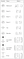A representative set of quantum gates for universal computation. Source: QCAD, a quantum computer simulation program.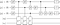A sample quantum circuit diagram with gates applied to a series of qubits, image via QASM software for visualizing quantum circuits. Note that the qubits start on the left hand side in a classical state, then proceed to the right through various quantum gates, with the dial figures on right side represent the measurements of qubits.

The ability to achieve a given type of quantum gate may be one of the defining feature of various quantum computer architectures. For example the D-Wave architecture is a single purpose quantum annealing machine for optimization problems and does not have a sufficient set of gates for universal quantum computation. The approach employed by D Wave is known as Adiabatic quantum computation - this approach is different than the quantum circuit model that we are discussing here, hence the “controversy” as to whether D Wave will ever achieve actual asymptotic speedup (scaling with larger inputs) as opposed to constant factor speedup vs. classical architectures running simulated annealing — the classic computer’s alternate to quantum annealing.

It is fairly straight forward to picture the results of classical gates. Bits are either flipped or combined in some fashion from an input of 1’s and 0’s to an output of 1’s and 0’s. For quantum gates, the gate’s manipulation of the state is a little more conceptually abstract because we are not directly manipulating the qubit state, only the superposition’s probability of a measurement revealing a given state, and this is where the bloch sphere visualization for quantum superposition really comes in handy.

Note that for the graphical representation of a two qubit system simply displaying two separate bloch spheres will not be sufficient, we would also need some way to capture any entanglement between the two qubits. Entanglement is actually an important feature unique to quantum mechanics, computation, and information deserving a little more attention so I will take this opportunity to go off on a tangent. A primary and simple example of two entangled qubits is known as the Bell State represented as ψ=(|00>+|11>)/√2, where using the same derivation of probability trick as described above we find that there is a 50% probability of both qubits being found in the 0 state, a 50% chance of both qubits being found in the 1 state, and a 0% probability of the qubits being found in opposite states such as |01> or |10>. One way to prepare a Bell state is to take two qubits both initialized to |0>, then apply a Haldamard gate to one and CNOT gate as shown below. Once we achieve the Bell State, if we measure one of the the two qubits we will immediately know the state of the other — which might come in handy if we want to test the EPR Paradox.

Just like in a classical computer, there exists a set of quantum gates that allow us to simulate any reversible quantum circuit aka a universal quantum gate set. This gate set can be achieved with the CNOT and single qubit unitary gates, or alternatively with multi qubit gates such as a quantum analog to the Fredkin gate.

3. How does a quantum computer work?

Having laid the groundwork of building blocks for computation such as qubits, error correction, gates, and entanglement, I think we’re finally ready to dive into the actual operation of a quantum computation. It’s actually pretty simple:

A quantum computation starts with initialized qubits, and then a series of unitary transformations are applied to transform the system into the desired configuration of superposition and entanglement — these series of gates are how we actualize a quantum algorithm — more on algorithms to follow. Once the series of gates and transformations are applied measurements are taken of the qubits. An important distinction between quantum and classical computers is that a quantum computer is probabilistic, thus these measurements of algorithmic outputs only give the proper solution within an algorithm specific confidence interval. The computation is then repeated until satisfactory probable certainty of solution can be achieved. The repetition of computation is necessary because of the no-cloning theorem — although a quantum state can be teleported, in so doing the original source must be measured, thus a quantum state cannot be copied or even converted into digital bits.

4. What can a quantum computer do?

The answer to what a quantum computer enables that can’t be achieved with a classical computer goes hand in hand with a discussion of quantum algorithms — those algorithms which are only possible with the quantum model of computation. First it’s worth debunking a potential misconception. The exponential size of quantum state space sometimes causes speculation that a quantum computer could provide exponential speedup for all computations — a claim that sometimes even makes it into popular press accounts. This is unfortunately not the case, while there are specific applications that can achieve some speedup, they are limited to those enabling algorithms. So when we say that quantum computers will take us outside of Moore’s Law, that is really only accurate for some specific applications as enabled by quantum algorithms. Thus the quantum computing race is as much to build robust / scalable / low error qubit architecture as it is to design novel algorithms.

Before diving into specifics of of algorithms and applications, probably worth at least briefly first climbing up a few layers of abstraction for a discussion of the theory of computational complexity. Computational complexity theory is a way of categorizing various styles of computer problems into classes based on how hard it is for a computer to solve them (I am definitely oversimplifying here, this is my layman’s description). Shown below are the most important major categories. P (Polynomial) represents the category of problems that can be solved by a classical computer in polynomial time, or in other words these are the problems that you can handle on your laptop. As we get higher up in this scale the amount of computational power and time required grows to data center scale and then even beyond those capabilities. A key category relevant for quantum computers is BQP (Bounded Quantum Polynomial) which represents the class of problems that can be solved by a quantum computer in polynomial time. In other words, quantum computers enlarge the class of problems that can be solved in polynomial time from P to BQP (which encompasses P). It’s also worth noting that the actual shape of the BQP class in relation to the others shown is not known for sure, it is possible that BQP is larger than what is shown here but until such time that we discover quantum algorithms that actually demonstrate this then we are left to speculate. (People have long argued over the possibility of whether P could actually be equal to NP and many an arm-chair Turing has tried to prove P=NP to no avail, perhaps an eventual discovery of BQP=NP is somewhat more realistic although the optimality of Grover’s search algorithm suggests that this is likely not the case.)

This image shown is a broad overview of key categories, if you want to get down to the nitty gritty sub-classes and categories there is a (intentionally not using the work “useful”) reference available online. I don’t recommend trying to wade through this level of detail, mainly providing that link for completeness and because it looks like someone may have put a lot of work into its assembly. Another aside is that one shouldn’t confuse computational complexity with the more general complexity studies as one may encounter at Santa Fe Institute for instance, although it is perhaps interesting to note that the two fields do have potential for intersections.

Here now are a few examples of the type of applications and algorithms that quantum computers will enable. Many of these algorithms will have some equivalent in classical architecture, with the quantum computer providing some degree of speedup, others, such as a true randomness generation or the entanglement enabling of quantum information systems, may have no equivalent in classical architecture.

a) Randomness: it is a limitation and a challenge of classical computers to achieve true randomness. In applications such as cryptography an attacker who can predict certain values can potentially view an encoded message. Classical systems have attempted to generate a pseudo randomness using pseudo random inputs, for example Mathematica long used Cellular Automata Rule 30 coupled with the inputs of some computer state to generate a pseudo randomness — although I believe they have since switched to a new approach. The Rand Corporation published some of the earliest collections of random numbers in book format (the Amazon reviews are priceless). A quantum computer’s true randomness generated from the collapse of a qubit’s quantum state makes for more robust cryptography and also comes in handy for other applications such as Monte Carlo analysis or computer simulations of real world systems.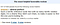An Amazon user’s review of “A Million Random Digits with 100,000 Normal Deviates”

b) Search: One style of quantum algorithms are built with a technique of in possible answer superposition, the quantum state is manipulated so that incorrect answers interfere with each other and the solution is reinforced, a key example of this technique is found in Grover’s Algorithm, an algorithm that provides (only) quadratic speedup for applications in database search. With all of the talk about Google diving into the quantum computer field, purchasing D Wave machines, etc., one may expect that this search application is a primary focus, but I believe their interests are more geared towards applications in artificial intelligence.

c) Factoring: Some quantum algorithms make use of the Quantum Fourier transform in their operation. The Fourier transform, to those uninitiated, is a method for taking a noisy signal and breaking it down into its constitute frequencies and amplitudes, sort of analogous to taking a smoothie and running through a series of filters to determine ingredients.

The Shor’s factoring algorithm is a good example of applications that make use of this effect. Used for integer factoring, the breaking down of numbers into their constituent prime multipliers, which has long been the key ingredient for RSA cryptography (still widely used for securing communications). Factoring was chosen for this application because there is no classical algorithm which is known to solve this puzzle in polynomial time, thus for large enough integers the problem of factoring even with considerable classical resources can take exponential time (read years / decades even with data centers scale computational capacity), making this cryptography protocol secure. The advent of quantum computers employing Shor’s algorithm will change that. If you think the few email leaks we are seeing in this presidential election are bad, just wait until modern cryptography becomes obsolete, then those who have been monitoring and storing decades of encrypted communications may just have the ability to read them at will. Fortunately there are alternatives to RSA encryption such as quantum encryption methods, which are secure even in a world with quantum computers. The transition of our communication systems from RSA style cryptography protocols to those more secure I speculate could turn into a challenge on par with the Y2K Bug from the turn of the millennium (as immortalized in the movie Office Space) — perhaps a “Q2K”?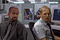No one in this country can ever pronounce my name right. It’s not that hard: Na-ghee-na-na-jar. Nagheenanajar. (image source)

d) Optimization: I’ve already touched on the concept of quantum annealing with respect to the D Wave architecture, and won’t spend much more time on it here other than to just reiterate the broad applications of improved optimization techniques enabled by the tunneling of quantum annealing.

Optimization has wide applications. For some more outlandish consider some talk of using quantum annealers to model software systems to minimize errors and bugs. Others may have focus on applications in space exploration. The philosopher Nassim Taleb has even cautioned on the overuse of optimization due to a tendency to inflate hidden risks / increase the fragility of a system.

e) The zoo: For a complete list of current publicly known quantum algorithms NIST has assembled a menagerie here.

5. The types of quantum computers.

This post is getting a little long, so I’ll try to wrap things up with a brief comment on the different types of quantum computers. It’s worth repeating that the machine that has been longest on the market, the D Wave, is not a general purpose computer, it is a specialized machine with single purpose use of quantum annealing. Whether their scientists will ever be able to map the gates of a universal quantum gate set onto their annealing architecture may still be a possibility but it is not there yet. Another player you may have heard of has recently come forth with a successful multi-qubit architecture, this time IBM. What’s more, they actually are making that architecture available to the general public complete with tutorials, simulations, and actual quantum computing cycles through their Quantum Experience. (Cool!) For those with any interest in learning more about quantum computers and their algorithms this experience is probably a good next step.

Books that were referenced here or otherwise inspired this post:

## Quantum Computation and Quantum Information — Michael Nielsen and Isaac Chuang

(As an Amazon Associate I earn from qualifying purchases.)

Albums that were referenced here or otherwise inspired this post:

## Fresh Mode — Ugly Duckling

As an Amazon Associate I earn from qualifying purchases.

If you enjoyed or got some value from this post please consider paying it forward and documenting some of your interests as well — Wikipedia is always accepting submissions! Or I don’t know, consider hiring me or something. :)

Written by

## From the Diaries of John Henry

#### Essays and Explorations

Welcome to a place where words matter. On Medium, smart voices and original ideas take center stage - with no ads in sight. Watch
Follow all the topics you care about, and we’ll deliver the best stories for you to your homepage and inbox. Explore
Get unlimited access to the best stories on Medium — and support writers while you’re at it. Just \$5/month. Upgrade